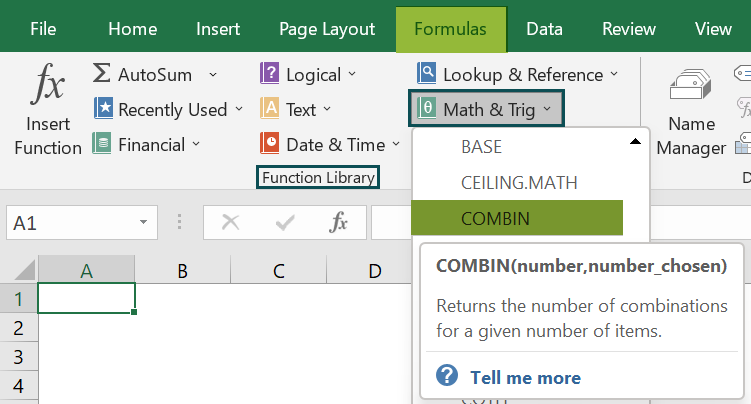# COMBIN Function In ExcelArticle byExcelMojo Team## What Is COMBIN Function In Excel?

The COMBIN function in Excel helps users estimate the number of combinations or possible groups that can be formed from a dataset. The order of the selected values doesn’t matter, and repetitions are not allowed by the function. The COMBIN function, from the Math & Trig group, is an inbuilt function, so we can insert the formula from the “Function Library” or enter it directly in the worksheet.

For example, we will apply the COMBIN function in Excel to calculate the combination values of the given numbers.

Select cell A2, enter the formula =COMBIN(3,2), and press “Enter.” The result is ‘3,’ as shown above, i.e., the combination of ‘a,’ ‘b,’ & ‘c’ in 2 digits.

###### Key Takeaways
• The COMBIN function in Excel is a mathematical function that returns the combination of data values.
• If the arguments number and number_chosen are decimal values, then the function only considers the whole integer.
• Excel COMBINA and COMBIN functions both calculate combinations of a set of objects or several items. The difference between these functions is the COMBINA function counts repetitions of combinations, whereas the COMBIN function does not count the repetitions.
• When we have a list with duplicate entries, the function will give incorrect results, as the function only counts unique entries.

### COMBIN() Excel Formula

The syntax of the COMBIN Excel formula is,

The arguments of the COMBIN Excel formula are,

• number: It is a mandatory argument. It is the cell value to find the combination, which has to be greater than or equal to 0 and the number_chosen.
• number_chosen: It is a mandatory argument. It is the number of groups/digits to be formed in each combination. It is always a number and should be greater than or equal to 0.

### How To Use COMBIN Excel Function?

We can use the COMBIN Excel function in 2 ways, namely,

1. Access from the Excel ribbon.
2. Enter in the worksheet manually.

#### Method #1 – Access from the Excel ribbon

First, choose an empty cell → select the “Formulas” tab → go to the “Function Library” group → click the “Math & Trig” option drop-down → select the “COMBIN” function, as shown below.

The “Function Arguments” window appears. Enter the argument values in the “Number”, and “Number_chosen” fields → click “OK”, as shown below.

#### Method #2 – Enter in the worksheet manually

1. Select an empty cell for the output.
2. Type =COMBIN( in the selected cell. [Alternatively, type “=C” and double-click the COMBIN function from the list of suggestions shown by Excel.]
3. Enter the arguments as cell values or cell references and close the brackets.
4. Press the “Enter” key.

Let us understand this function with a basic example.

We will calculate the combination of numbers of the given data using COMBIN function in Excel.

In the table, the data is,

• Column A: contains Values.
• Column B: contains Output.

The steps to calculate the combination using COMBIN function in Excel are,

1. Select cell B2, and enter the formula =COMBIN(5, i.e., 5 is the number value.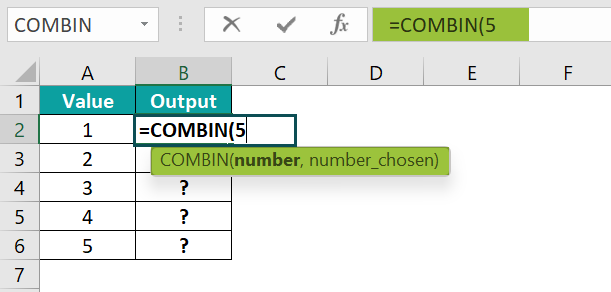2. In continuation with the formula, enter the value of ‘number_chosen’ as “A2”, and close the brackets. The complete formula is =COMBIN(5,A2).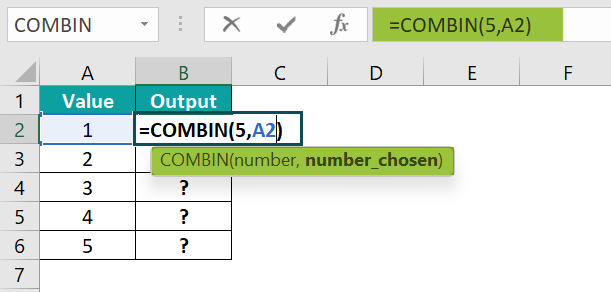3. Press the “Enter” key. The result is 5, as shown below.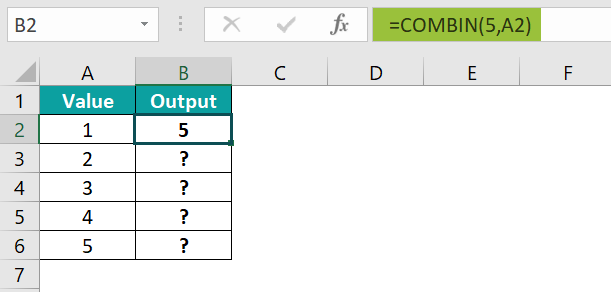4. Drag the formula from cell B2 to B6 using the fill handle.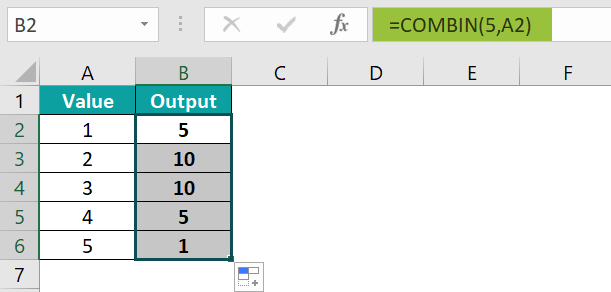The output is shown above.

### Examples

We will understand some advanced scenarios using the COMBIN function in Excel examples.

#### Example #1

We will calculate the combination of numbers of the given data using the COMBIN function in Excel. In the table, the data is,

• Column A: contains the Result.

The procedure to calculate the combination using the COMBIN function is,

• Step 1: Select cell A2, enter the formula =COMBIN(15,2), and press the “Enter” key.

The result is “105”, as shown above.

#### Example #2

We will calculate the combination of numbers of the given data using the COMBIN function.

In the table, the data is,

• Column A: contains Values.
• Column B: contains Output.

The procedure to calculate the combination using the COMBIN function in Excel is,

• Step 1: Select cell B2, enter the formula =COMBIN(A2,A3), and press the “Enter” key.

The result is “252”, as shown above.

#### Example #3

We will calculate the combination of numbers of the given data using the COMBIN function in Excel.

In the table, the data is,

• Column A: contains the Number.
• Column B: contains Number_chosen.

The steps to calculate the combination using the COMBIN function are,

• Step 1:  Select cell C2, enter the formula =COMBIN(A2,B2), and press the “Enter” key. The result is “45”, as shown below.
• Step 2: Drag the formula from cell C2 to C3 using the fill handle.

The output is shown above.

### Important Things to Note

• The #VALUE! error occurs if the number and number_chosen arguments are cell references with non-numeric values.
• The #NAME? error occurs if the number and number_chosen arguments are non-numeric values.
• The #NUM! error occurs when the value or argument is outside its constraint.
• The input number argument is less than 0 or a negative number.
• The input number_chosen argument is less than 0 or is greater than the number argument.

1. What does the COMBIN function do in Excel?

The COMBIN function in Excel, aka the combination function, calculates the number of possible combinations for two given numbers.

The syntax of the COMBIN function is =COMBIN(number,number_chosen)

2. How does the COMBIN function work in Excel?

The COMBIN function in Excel works in Excel as follows:

1.     Select an empty cell for the output.
2.     Type =COMBIN( in the selected cell. [Alternatively, type “=C” and double-click the COMBIN function from the list of suggestions shown by Excel.]
3.     Enter the arguments as cell values or cell references and close the brackets.
4.     Press the “Enter” key.

For example, we will use the COMBIN function to calculate the combination of the following data.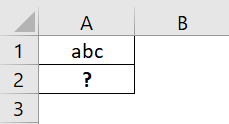Select cell A2, enter the value =COMBIN(3,3), and press the “Enter” key.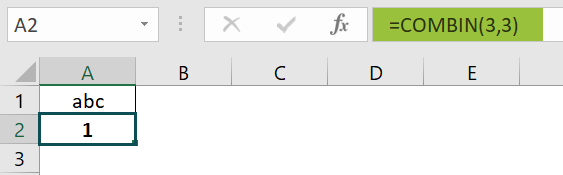The result is “1”, as shown above.

3. Where is the COMBIN function in Excel?

The COMBIN function in Excel is in the “Formulas” tab and can be inserted as follows:

First, choose an empty cell → select the “Formulas” tab → go to the “Function Library” group → click the “Math & Trig” option drop-down → select the “COMBIN” function, as shown below.